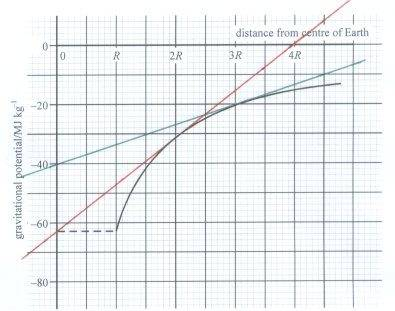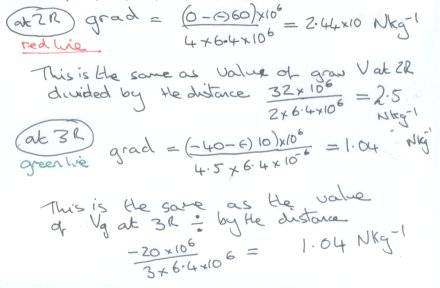# Field strength is the potential gradient graph question

mrcotton

## Homework Statement

By use of the graph in part (a), calculate the gravitational field strength at a distance 2R from the centre of the Earth.

## Homework Equations

g = delta V / Delta r filed strength is the potential gradient## The Attempt at a Solution

I have drawn the gradient on at 2r=R and calculated it. I have also done another at 3R to check what is confusing me.I've just written out the equations; if V=GM/r and you divide that by r you get GM/r^2, which = g. So this must be valid for any point. In that case, why bother with the inaccuracy of drawing a tangent? Why not just read off the value of V at that value of r??

D

Mentor
If you know the exact shape of the potential everywhere, you can calculate the gradient, of course.
In general, this does not have to be true. Inside earth, for example, force and potential are more complicated functions.

mrcotton
Thanks mfb for responding.
So will the potential at any point divided by the distance to that point always equal the gravitational field strength or is this because my potential function has this shape?

mrcotton
So if

g = \frac{\Delta V}{\Delta r}

Can why can we say that V at that point divided by the distance is equal to g?

mrcotton
hmm, looks like I need to add something to the LATEX statement so it is parsed correctly?

Mentor
Thanks mfb for responding.
So will the potential at any point divided by the distance to that point always equal the gravitational field strength or is this because my potential function has this shape?
It works if you have a single, spherical symmetric mass at the origin (in 3 dimensions), and consider the potential and force outside this mass, where the potential is defined to be zero at infinite distance.
It fails as soon as one of those conditions is not true.

You need $$code$$ or $code$ to parse TeX (you can also use the shorter versions $$code$$ and ##code##).

mrcotton
Thanks again mfb
so in my particular case it works for the potential GM/r
but the general case is $$g = \frac{\Delta V}{\Delta r}$$ is always true.
If this LATEX works then I have learn't two new things today

Now to go and learn the functions for the potentials for the standard model Lagrangian
D